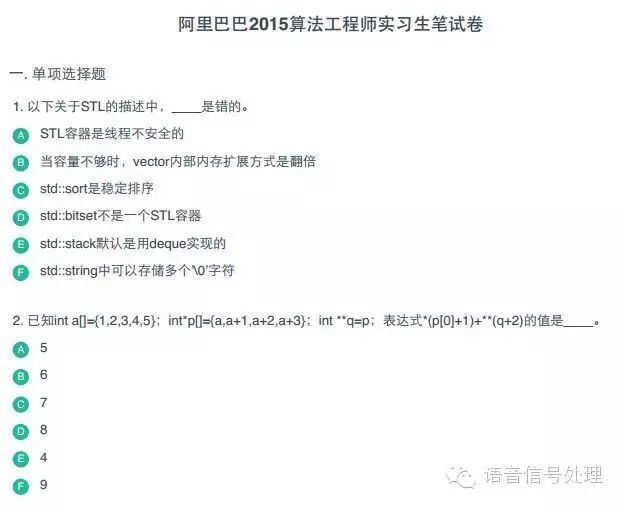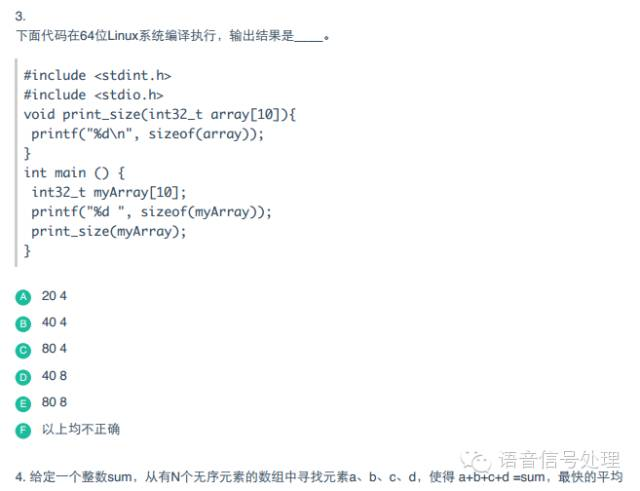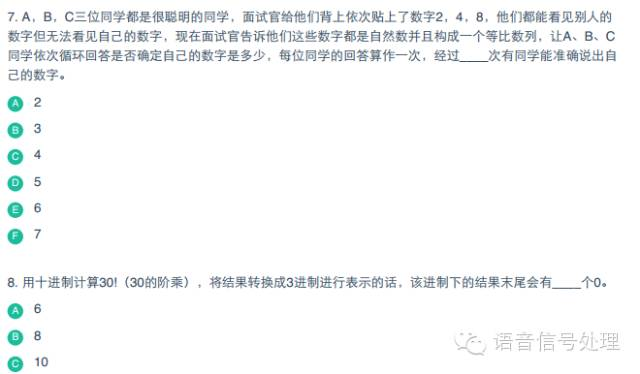# 阿里腾讯面试一二# 阿里巴巴算法、数据工程师笔试题选解

1、有三个结点的，可以构成多少个种叉树？

2、一副牌52张(去掉大小王)，从中抽取两张牌，一红一黑的概率是多少？

3设计一个最优算法来查找一n个元素数组中的最大值和最小值。已知一种需要比较2n次的方法，请给一个更优的算法。情特别注意优化时间复杂度的常数。

4已知三个升序整数数组a[l], b[m]和c[n]。请在三个数组中各找一个元素，是的组成的三元组距离最小。三元组的距离定义是：假设a[i]、b[j]和c[k]是一个三元组，那么距离为:

Distance = max(|a[ I ] – b[ j ]|, |a[ I ] – c[ k ]|, |b[ j ] – c[ k ]|)

5在黑板上写下50个数字：1至50.在接下来的49轮操作中，每次做如下动作：选取两个黑板上的数字a和b，擦去，在黑板上写|b – a|。请问最后一次动作之后剩下数字可能是什么？为什么？1、有三个结点的，可以构成多少个种树形结构？2、一副牌52张(去掉大小王)，从中抽取两张牌，一红一黑的概率是多少？

P = [C(1,26) * C(1,26) ] / C(2,52) = 26 * 26 / (26 * 51) = 26/51

P = 1 – C(2,26) / C(2,52) – C(2,26) / C(2,52) = 1 – 2 * (26 * 25)/(51 * 52) = 1 – 25/51 = 26/51

3设计一个最优算法来查找一n个元素数组中的最大值和最小值。已知一种需要比较2n次的方法，请给一个更优的算法。情特别注意优化时间复杂度的常数。

N/2 * 3 = (3N)/2 次#include <stdio.h>
#include <stdlib.h>
#define N 7
int main()
{
int arr[N] = {4, 1, 5, 9, 9, 7, 10};
int iter = 0;
int cnt = 0;
for(iter = 0; iter <= N / 2 + 1 ; iter += 2)
{
if(++cnt && arr[iter] > arr[iter + 1] )
{
int temp = arr[iter];
arr[iter] = arr[iter + 1];
arr[iter + 1] = temp;
}
}
int myMin = arr;
for(iter = 2; iter < N ; iter += 2)
{
if(++cnt && arr[iter] < myMin)
{
myMin = arr[iter];
}
}
int myMax = arr;
for(iter = 3; iter < N; iter += 2)
{
if(++cnt && arr[iter] > myMax)
{
myMax = arr[iter];
}
}
if(N % 2 != 0 && ++cnt && myMax < arr[N - 1]) myMax = arr[N - 1];
printf("min is %d\n", myMin);
printf("max is %d\n", myMax);
printf("compare times is %d", cnt);
return 0;
}4已知三个升序整数数组a[l], b[m]和c[n]。请在三个数组中各找一个元素，是的组成的三元组距离最小。三元组的距离定义是：假设a[i]、b[j]和c[k]是一个三元组，那么距离为:

Distance = max(|a[ I ] – b[ j ]|, |a[ I ] – c[ k ]|, |b[ j ] – c[ k ]|)

Distance = max(|x1 – x2|, |x1 – x3|, |x2 – x3|) = max(   max(|x1 – x2|, |x1 – x3|) , |x2 – x3|)   –公式（2）

根据公式（1），max(|x1 – x2|, |x1 – x3|) = 1/2 ( |2x1 – x2– x3| +  |x2 – x3|)，带入公式（2），得到

Distance = max( 1/2 ( |2x1 – x2– x3| +  |x2 – x3|) , |x2 – x3| )

=1/2 * max(  |2x1 – x2– x3|  , |x2 – x3| ) + 1/2*|x2 – x3| //把相同部分1/2*|x2 – x3|分离出来

=1/2 * max(  |2x1 – (x2 + x3)|  , |x2 – x3| ) + 1/2*|x2 – x3|   //把(x2 + x3)看成一个整体，使用公式（1）

=1/2 * 1/2 *((|2x1 – 2x2| + |2x1 – 2x3|) + 1/2*|x2 – x3|

=1/2 *|x1 – x2| + 1/2 * |x1 – x3| + 1/2*|x2 – x3|

=1/2 *(|x1 – x2| + |x1 – x3| + |x2 – x3|)  //求出来了等价公式，完毕！

第二个关键点：如何找到(|x1 – x2| + |x1 – x3| + |x2 – x3|) 的最小值，x1，x2，x3，分别是三个数组中的任意一个数，这一题，我只是做到了上面的推导，后面的算法设计是由csdn上的两个朋友想出来的方法，他们的CSDN的ID分别为 “云梦泽” 和 “shuyechengying ”.

用三个指针分别指向a,b,c中最小的数，计算一次他们最大距离的Distance ，然后在移动三个数中较小的数组指针，再计算一次，每次移动一个，直到其中一个数组结束为止，最慢(l+ m + n)次，复杂度为O(l+ m + n)#include <stdio.h>
#include <stdlib.h>
#include <math.h>
#define l 3
#define m 4
#define n 6
int Mymin(int a, int b, int c)
{
int Min = a < b ? a : b;
Min = Min < c ? Min : c;
return Min;
}

int Solvingviolence(int a[], int b[], int c[])
{
//暴力解法，大家都会，不用过多介绍了！
int i = 0, j = 0, k = 0;
int MinSum = (abs(a[i] - b[j]) + abs(a[i] - c[k]) + abs(b[j] - c[k])) / 2;
//    int store = {0};
int Sum = 0;
for(i = 0; i < l; i++)
{
for(j = 0; j < m; j++)
{
for(k = 0; k < n; k++)
{
Sum = (abs(a[i] - b[j]) + abs(a[i] - c[k]) + abs(b[j] - c[k])) / 2;
if(MinSum > Sum)
{
MinSum = Sum;
//                    store = i;
//                    store = j;
//                    store = k;
}
}
}
}
//    printf("the min is %d\n", minABC);
//    printf("the three number is %-3d%-3d%-3d\n", a[store], b[store], c[store]);
return MinSum;

}

int MinDistance(int a[], int b[], int c[])
{
int MinSum = 0; //最小的绝对值和
int Sum = 0;  //计算三个绝对值的和，与最小值做比较
int MinOFabc = 0; // a[i] , b[j] ,c[k]的最小值
int cnt = 0;  //循环次数统计，最多是l + m + n次
int i = 0, j = 0, k = 0;  //a,b,c三个数组的下标索引
MinSum = (abs(a[i] - b[j]) + abs(a[i] - c[k]) + abs(b[j] - c[k])) / 2;
for(cnt = 0; cnt <= l + m + n; cnt++)
{
Sum = (abs(a[i] - b[j]) + abs(a[i] - c[k]) + abs(b[j] - c[k])) / 2;
MinSum = MinSum < Sum ? MinSum : Sum;
MinOFabc = Mymin(a[i] ,b[j] ,c[k]);//找到a[i] ,b[j] ,c[k]的最小值
//判断哪个是最小值，做相应的索引移动
if(MinOFabc == a[i])
{
if(++i >= l) break;
}//a[i]最小,移动i

if(MinOFabc == b[j])
{
if(++j >= m) break;
}//b[j]最小,移动j
if(MinOFabc == c[k])
{
if(++k >= n) break;
}//c[k]最小,移动k

}
return MinSum;
}
int main(void)
{
int a[l] = {5, 6, 7};
int b[m] = {13, 14, 15, 17};
int c[n] = {19, 22, 24, 29, 32, 42};

printf("\nBy violent solution ,the min is %d\n", Solvingviolence(a, b, c));
printf("\nBy Optimal solution ,the min is %d\n", MinDistance(a, b, c));
return 0;
}
posted @ 2016-05-19 21:55  Code~Rush  阅读(902)  评论(0编辑  收藏  举报